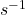## What is the wavelength of a 340-Hz sound wave when the speed of the sound in air is 340 m/s?

Question

What is the wavelength of a 340-Hz sound wave when the speed of the sound in air is 340
m/s?

in progress 0
1 month 2021-08-13T20:45:40+00:00 1 Answers 2 views 0

Wavelength of sound wave = 1 m

Explanation:

Given

speed of sound wave = 340 mfrequency of sound wave = 340 Hz = 340wavelength of sound wave = ?

We know that for all waves, the relationship between wavelength λ, frequency f and speed v is same and given by the equation:

v = fλ

Hence wavelength of sound wave λ = v/f

=  340 m/ 340= 1 m